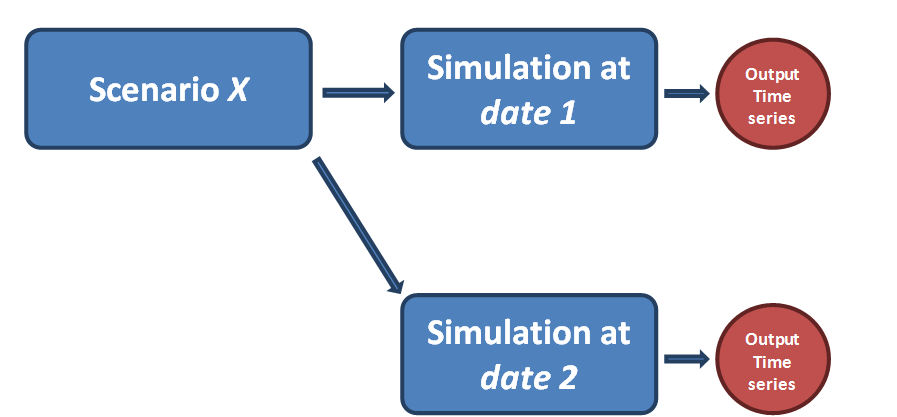Model setup (or model): A Numerical model developed for the problem(s) to be analyzed.

Scenario: Possible existing or future situations that need to be analyzed.

Simulation: A time-varying description of certain behavior of the natural system as computed by the mathematical model. A simulation will produce outputs referred to as simulation results.

Model Setups, Scenarios and Simulations linkage in DSS

Figure 1 shows the relation between a model setup and scenarios.Figure 1: Model setup and scenarios

A model setup is a model that is built, checked and run successfully outside the DSS using a modeling tool (e.g. Mike hydro or Mike 11). Once this is done, this model setup can be registered (imported) into the DSS. Any number of scenarios can be created based on each model setup (See Figure 1). Those scenarios are based on the same model setup but vary in model parameters or input data (e.g. catchment inflow time series) and in the selected outputs configured. If model setup changes (i.e. through adding additional model objects) are needed, this needs to be done again outside the DSS using the same or a compatible modeling tool to make the changes, verify them and run the model successfully. Following this, the new model setup can be registered in the DSS and can have its own scenarios.

Each scenario in the DSS needs to run to obtain results. Each scenario run is called a 'Simulation' as shown in Figure 2. For each simulation, outputs, configured when creating the scenario, are created and stored in the database. If at a later time, changes are made to the same scenario. When it is run a new simulation is created with new outputs. Unlike most modeling tools, only outputs that are selected when the scenario is configured are saved. This is to optimize the database size, especially for large models.Figure 2: Scenario and simulations